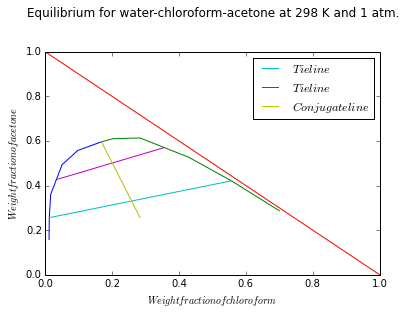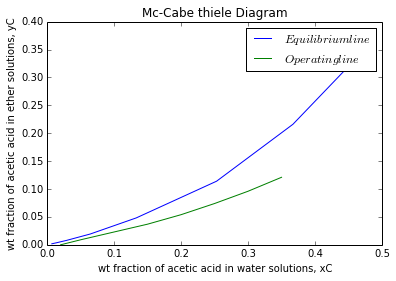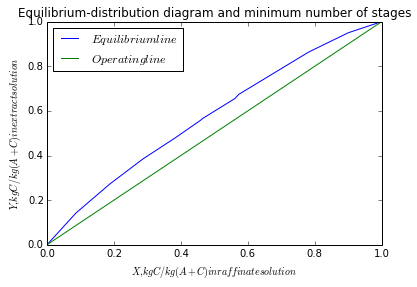# Chapter 7:Liquid-Liquid Extraction¶

### Example 7.1,Page number:429¶

In :
from pylab import *

#For water phase:
Cw=[1.23,1.29,1.71,5.10,9.8,16.90]        #Chloroformm concentration in wt %
Aw=[15.80,25.6,36.0,49.30,55.7,59.60]     #Acetone concentration in wt %

#For Chloroform phase
Cc=[70.0,55.7,42.9,28.4,20.4,16.9]       #Chloroformm concentration in wt%
Ac=[28.70,42.10,52.70,61.30,61.00,59.6]   #Acetone concentration in wt %
for i in range(0,6):
Cw[i]=Cw[i]/100            #Weight fraction
Aw[i]=Aw[i]/100            #Weight fraction
Cc[i]=Cc[i]/100            #Weight fraction
Ac[i]=Ac[i]/100            #Weight fraction

a1=plot(Cw,Aw)
a2=plot(Cc,Ac)
X=linspace(1,0)
Y=linspace(0,1)
a3=plot(X,Y)
xlabel("$Weight fraction of chloroform$")
ylabel("$Weight fraction of acetone$")
title("Equilibrium for water-chloroform-acetone at 298 K and 1 atm.\n\n")
a4=plot([Cw,Cc],[Aw,Ac],label='$Tie line$')
a5=plot([(Cw+Cw)/2,(Cc+Cc)/2],[(Aw+Aw)/2,(Ac+Ac)/2],label='$Tie line$')
a6=plot([Cw,Cc],[Aw,Aw],label='$Conjugate line$')
legend(loc='upper right')

Out:
<matplotlib.legend.Legend at 0x7a98978>### Example 7.2,Page number:433¶

In :
#Variable declaration
#  'b'-solvent   'f'-feed   'r'-raffinate   'e'-extract   'c'-one of the   # component in 	 feed
F = 50  					# [feed rate, kg/h]
S = 50  					# [solvent rate, kg/h]
xcf = 0.6
xbf = 0
ycs = 0
ybs = 1.0
# The equilibrium data for this system can be obtained from Table 7.1 and    # Figure 7.6
# Plot streams F (xcF = 0.6, xBF = 0.0) and S (yes = 0.0, yBs = 1.0). After  # locating 	streams F and S, M is on the line FS  its exact location is found # by calculating xcm 	from
#Calculation

xcm = (F*xcf+S*ycs)/(F+S)

# From figure 7.8
xcr = 0.189
xbr = 0.013
yce = 0.334
ybe = 0.648
M = F+S  					# [kg/h]
# From equation 7.8
E = M*(xcm-xcr)/(yce-xcr)  			# [kg/h]
R = M-E  					# [kg/h]

#Result
print"The extract and raffinate flow rates are",round(E,2),"kg/h and",round(R,2)," kg/h respectively\n"

print"The compositions when one equilibrium stage is used for the separation is",xcr,"and",xbr," in raffinate phase for component b and c respectively and",yce,"and",ybe,"in extract phase for component b and c respectively"

The extract and raffinate flow rates are 76.55 kg/h and 23.45  kg/h respectively

The compositions when one equilibrium stage is used for the separation is 0.189 and 0.013  in raffinate phase for component b and c respectively and 0.334 and 0.648 in extract phase for component b and c respectively


### Example 7.4,Page number:439¶

In :
#Variable declaration

# C-acetic acid    A-water
# f-feed   r-raffinate   s-solvent
fd = 1000  						# [kg/h]
xCf = 0.35 						# [fraction of acid]
xAf = 1-xCf  						# [fraction of water]
# Solvent is pure
xAr = 0.02
yCs = 0

import math
from pylab import *
from numpy import *
print "Solution 7.4(a)\n"
# Solution(a)

# From Figure 7.15
xCMmin = 0.144
# From equation 7.11
Smin = fd*(xCMmin-xCf)/(yCs-xCMmin)  				# [kg/h]

#result
print"The minimum amount of solvent which can be used is",round(Smin),"kg/h"

print "Solution7.4(b)"
# Solution(b)

S = 1.6*Smin  							# [kg/h]
# From equation 7.11
xCM = (fd*xCf+S*yCs)/(fd+S)

# Data for equilibrium line
# Data_eqml = [xCeq yCeq]
Data_eqml =matrix([[0.0069,0.0018],[0.0141,0.0037],[0.0289,0.0079],[0.0642,0.0193],[0.1330,0.0482],[0.2530,0.1140],[0.3670,0.2160],[0.4430,0.3110],[0.4640,0.3620]])

# Data for operating line
# Data_opl = [xCop yCop]
Data_opl =matrix([[0.02,0],[0.05,0.009],[0.1,0.023],[0.15,0.037],[0.20,0.054],[0.25,0.074],[0.30,0.096],[0.35,0.121]])

a1=plot(Data_eqml[:,0],Data_eqml[:,1],label='$Equilibrium line$')
a2=plot(Data_opl[:,0],Data_opl[:,1],label='$Operating line$')
legend(loc='upper right')
xlabel("wt fraction of acetic acid in water solutions, xC")
ylabel("wt fraction of acetic acid in ether solutions, yC")
title('Mc-Cabe thiele Diagram')

# Now number of theoritical stages is determined by drawing step by step  # stairs from	 	xC = 0.35 to xC = 0.02
# From figure 7.16
# Number of theoritical stages 'N' is
N = 8

show(a1)
show(a2)
print"\nThe number of theoretical stages if the solvent rate used is 60 percent above the minimum is ",N

Solution 7.4(a)

The minimum amount of solvent which can be used is 1431.0 kg/h
Solution7.4(b)The number of theoretical stages if the solvent rate used is 60 percent above the minimum is  8


### Example 7.5,Page number:444¶

In :
#Variable declaration

# C-nicotine    A-water    B-kerosene
# F-feed    R-raffinate    S-solvent
F = 1000  					# [feed rate, kg/h]
xAF = 0.99  					# [fraction of water in feed]
# Because the solutions are dilute therefore
xCF = 0.01 					# [fraction of nicotene in feed, kg nicotene/kg 						water]
xCR = 0.001  					# [fraction of nicotene in raffinate, kg 						nicotene/kg water ]
m = 0.926  					# [kg water/kg kerosene]
import math

print "Solution 7.5(a) "
# Solution(a)

yCS = 0  					# [kg nicotene/kg water]

# Because, in this case, both the equilibrium and operating lines are       # straight,if 	the minimum solvent flow rate Bmin is used, the concentration # of the exiting extract, 	yCmax, will be in equilibrium with xCF. Therefore
yCmax = m*xCF  # [kg nicotene/kg kerosene]

A = F*xAF  					# [kg water/h]
# From equation 7.17
Bmin = A*(xCF-xCR)/(yCmax-yCS)  		# [kg kerosene/h]

#Result
print"The minimum amount of solvent which can be used is",round(Bmin),"kerosene/h"

print"\nSolution 7.5(b)"
# Solution(b)

B = 1.2*Bmin  					# [kg kerosene/h]
EF = m*B/A
Nt = math.log((xCF-yCS/m)/(xCR-yCS/m)*(1-1/EF)+1/EF)/math.log(EF)

#Result

print"The number of theoretical stages if the solvent rate used is 20 percent above the minimum is",round(Nt,2)

print "Solution7.5(c)"
# Solution(c)

Eme = 0.6  					# [Murphree stage efficiency]
# from equation 7.20
Eo = math.log(1+Eme*(EF-1))/math.log(EF)  	# [overall efficiency]
Nr = Nt/Eo  					# [number of real stages]

#Result
print"The number of real stages required is",round(Nr)

Solution 7.5(a)
The minimum amount of solvent which can be used is 962.0 kerosene/h

Solution 7.5(b)
The number of theoretical stages if the solvent rate used is 20 percent above the minimum is 6.64
Solution7.5(c)
The number of real stages required is 11.0


### Example 7.6,Page number:449¶

In :
# C-styrene    A-ethylbenzene    B-diethylene glycol
F = 1000  # [kg/h]
XF = 0.6  # [wt fraction of styrene]
XPE = 0.9
XN = 0.1
# All above fractions are on solvent basis
# Equilibrium Data for Ethylbenzene (A)-Diethylene Glycol (B)-Styrene (C) at 298 K
# Data_eqm = [X Y]
# X - kg C/kg (A+C) in raffinate solution
# Y - kg C/kg (A+C) in extract solution
import math
from scipy.optimize import fsolve
from pylab import*
from numpy import*

Data_eqm=matrix([[0,0],[0.087,0.1429],[0.1883,0.273],[0.288,0.386],[0.384,0.48],[0.458,0.557],[0.464,0.565],[0.561,0.655],[0.573,0.674],[0.781,0.863],[0.9,0.95],[1,1]])

#Illustration 7.6(a)
# Solution(a)

# Minimum theoretical stages are determined on the XY equilibrium distribution diagram, stepping them off from the diagonal line to the equilibrium curve, beginning at XPE = 0.9 and ending at XN = 0.1

Data_opl=matrix([[0,0],[0.09,0.09],[0.18,0.18],[0.27,0.27],[0.36,0.36],[0.45,0.45],[0.54,0.54],[0.63,0.63],[0.72,0.72],[0.81,0.81],[0.90,0.90],[1,1]])

a1=plot(Data_eqm[:,0],Data_eqm[:,1],label='$Equilibrium line$')
a2=plot(Data_opl[:,0],Data_opl[:,1],label='$Operating line$')

legend(loc='upper left')
title('Equilibrium-distribution diagram and minimum number of stages')
xlabel("$X,kg C/kg (A+C) in raffinate solution$")
ylabel("$Y,kg C/kg (A+C) in extract solution$")

show(a1)
show(a2)
# Figure 7.20
Nmin = 9  # [number of ideal stages]

print"Ans.(a)The minimum number of theoretical stages are ",Nmin

#Illustration 7.6(b)
# Solution(b)

# Since the equilibrium-distribution curve is everywhere concave downward# ,the tie line 	which when extended passes through F provides the minimum
# reflux ratio
# From figure 7.19
NdeltaEm = 11.04
NE1 = 3.1
# From equation 7.30
# Y = R_O/P_E, external reflux ratio
Ymin = (NdeltaEm-NE1)/NE1  # [kg reflux/kg extract product]

print"Ans.(b)The minimum extract reflux ratio is",round(Ymin,3),"kg reflux/kg extract product"

#Illustration 7.6(c)
# Solution(c)

Y = 1.5*Ymin  # [kg reflux/kg extract product]
# From equation 7.30
NdeltaE = Y*NE1+NE1
# From figure 7.19
NdeltaR = -24.90
# From figure 7.21
N = 17.5  # [number of equilibrium stages]

# From figure 7.19
# For XN = 0.1 NRN = 0.0083
NRN = 0.0083
# Basis: 1 hour

# e = [P_E R_N]
# Solution of simultaneous equation
def G(e):
f1 = F-e-e
f2 = F*XF-e*XPE-e*XN
return(f1,f2)

# Initial guess:
e = [600,300]
y = fsolve(G,e)
P_E = y  # [kg/h]
R_N = y  # [kg/h]

R_O = Y*P_E  # [kg/h]
E_1 = R_O+P_E  # [kg/h]

B_E = E_1*NE1  # [kg/h]
E1 = B_E+E_1  # [kg/h]
RN = R_N*(1+NRN)  # [kg/h]
S = B_E+R_N*NRN  # [kg/h]

print"Ans(c.)The number of theoretical stages are",N
print"The important flow quantities at an extract reflux ratio of 1.5 times the minimum value are\n\n"
print"PE =",round(P_E),"kg/h\n","RN =",round(R_N),"kg/h\n","RO =",round(R_O),"kg/h\n","E1 =",round(E_1),"kg/h\n","BE =",round(B_E)," kg/h\n","E1 =",round(E1),"kg/h\n","RN =",round(RN),"kg/h\n","S =",round(S),"kg/h\n"Ans.(a)The minimum number of theoretical stages are  9
Ans.(b)The minimum extract reflux ratio is 2.561 kg reflux/kg extract product
Ans(c.)The number of theoretical stages are 17.5
The important flow quantities at an extract reflux ratio of 1.5 times the minimum value are

PE = 625.0 kg/h
RN = 375.0 kg/h
RO = 2401.0 kg/h
E1 = 3026.0 kg/h
BE = 9381.0  kg/h
E1 = 12407.0 kg/h
RN = 378.0 kg/h
S = 9384.0 kg/h



### Example 7.7,Page number:454¶

In :
#Variable declaration

Ff = 1.89  						# [cubic m/min]
Fs = 2.84  						# [cubic m/min]
t = 2  							# [min]

print "Solution 7.7(a)\n"
# Solution(a)
import math
Q = Ff+Fs  					# [total flow rate, cubic m/min]
Vt = Q*t  					# [cubic m]
# For a cylindrical vessel H = Dt
Dt = (4*Vt/math.pi)**(1.0/3.0)  		# [m]
H = Dt  					# [m]

#Result
print"The diameter and height of each mixing vessel is",round(Dt,1)," m and",round(H,1)," m respectively"

print"Solution 7.7(b) "
# Solution(b)
# Based on a recommendation of Flynn and Treybal (1955),
P = 0.788*Vt  					# [mixer power, kW]

#Result

print"The agitator power for each mixer is",round(P,2),"kW"

print"\nSolution 7.7(c)"
# Solution(c)

# Based on the recommendation by Ryan et al. (1959), the disengaging area  # in the 		settler is
# Dt1*L1 = Q/a = Y
a = 0.2 					# [cubic m/min-square m]
Y = Q/a  					# [square m]
# For L/Dt = 4
Dt1 = (Y/4)**0.5  				# [m]
L1 = 4*Dt1  					# [m]

#Result
print"The diameter and length of a settling vessel is",round(Dt1,2)," m and",round(L1,2)," m respectively"

print"Solution 7.7(d)"
# Solution(d)
# Total volume of settler
Vt1 = math.pi*Dt1**2*L1/4  			# [cubic m]
tres1 = Vt1/Q  					# [min]

#Result

print"The residence time in the settling vessel is",round(tres1,1),"min"

Solution 7.7(a)

The diameter and height of each mixing vessel is 2.3  m and 2.3  m respectively
Solution 7.7(b)
The agitator power for each mixer is 7.45 kW

Solution 7.7(c)
The diameter and length of a settling vessel is 2.43  m and 9.73  m respectively
Solution 7.7(d)
The residence time in the settling vessel is 9.5 min


### Example 7.8,Page number:456¶

In :
#Variable declaration

Ff = 1.61  					# [flow rate of feed, kg/s]
Fs = 2.24  					# [flow rate of solvent, kg/s]
t = 2*60  					# [residence time in each mixer, s]
df = 998  					# [density of feed, kg/cubic m]
uf = 0.89*10**-3  				# [viscosity of feed, kg/m.s]
ds = 868  					# [density of solvent, kg/cubic m]
us = 0.59*10**-3  				# [viscosity of solvent, kg/m.s]
sigma = 0.025  					# [interfacial tension, N/m]
g = 9.8  					# [square m/s]
import math

Qf = Ff/df  					# [volumetric flow rate of feed, cubic m/s]
Qs = Fs/ds  					# [volumetric flow rate of solvent, cubic m/s]
# Volume fractions in the combined feed and solvent entering the mixer
phiE = Qs/(Qs+Qf)
phiR = 1-phiE

print"\nSolution7.8(a)\n"
# Solution(a)
import math
Q = Qf+Qs  					# [total flow rate, cubic m/s]
Vt = Q*t  					# [vessel volume, cubic m]
# For a cylindrical vessel,  H = Dt
# Therefore,Vt = math.pi*Dt**3/4
Dt = (4*Vt/math.pi)**(1.0/3.0)  		# [ diameter, m]
H = Dt  					# [height, m]
Di = Dt/3  					# [m]

#Result
print"The height and diameter of the mixing vessel are",round(Dt,3)," m and",round(H,3)," m respectively.\n"
print"The diameter of the flat-blade impeller is",round(Di,3)," m"

print"\nSolution (b)\n"
# Solution(b)

# For the raffinate phase dispersed:
phiD = phiR
phiC = phiE
deltad = df-ds  				# [kg/cubic m]
rowM = phiD*df+phiC*ds  			# [kg/cubic m]
uM = us/phiC*(1 + 1.5*uf*phiD/(us+uf))  	# [kg/m.s]
# Substituting in equation 7.34

#Result

print"The minimum rate of rotation of the impeller for complete and uniform dispersion.is",round(ohm_min),"rpm."

print"\nSolution 7.8(c)"
# Solution(c)

ohm = 1.2*ohm_min  				# [rpm]

# From equation 7.37
Re = ohm/60*Di**2*rowM/uM  			# [Renoylds number]
# Then according to Laity and Treybal (1957), the power number, Po = 5.7
Po = 5.7
# From equation 7.37
P = Po*(ohm/60)**3*Di**5*rowM/1000  		# [kW]
# Power density
Pd = P/Vt  					# [kW/cubic m]

#Result
print"The power requirement of the agitator at 1.20 times the minimum rotation rate is",round(P,3)," kW."
print"Power density is",round(Pd,3),"kW/m^3"

Solution7.8(a)

The height and diameter of the mixing vessel are 0.862  m and 0.862  m respectively.

The diameter of the flat-blade impeller is 0.287  m

Solution (b)

The minimum rate of rotation of the impeller for complete and uniform dispersion.is 152.0 rpm.

Solution 7.8(c)
The power requirement of the agitator at 1.20 times the minimum rotation rate is 0.287  kW.
Power density is 0.571 kW/m^3


### Example 7.9,Page number:460¶

In :
#Variable declaration
# From example 7.8
Di = 0.288  					# [m]
sigma = 0.025  					# [N/m]
ohm = 152.0*1.2/60.0  				# [rps]
ds = 868.0  					# [kg/cubic m]
phiD = 0.385

#Calculation

import math
# Therefore from equation 7.49
We = Di**3*ohm**2.0*ds/sigma  			# [Weber number]

# From equation 7.50
dvs = Di*0.052*(We)**-0.6*math.exp(4*phiD)  	# [m]
# Substituting in equation 7.48
a = 6*phiD/dvs  				# [square m/cubic m]
dvs=dvs*1000					#[mm]
#Result
print"The Sauter mean drop diameter and the interfacial area is",round(dvs,3)," mm and",round(a)," square m/cubic m respectively."

The Sauter mean drop diameter and the interfacial area is 0.326  mm and 7081.0  square m/cubic m respectively.


### Example 7.10,Page number:461¶

In :
#Variable declaration

Dd = 1.15*10**-9  			# [molecular diffusivity of furfural in water, square 					m/s]
Dc = 2.15*10**-9  			# [molecular diffusivity of furfural in toluene, square 					m/s]
m = 10.15  				# [equilibrium distribution coefficient, cubic m 					raffinate/cubic m extract]

print"Solution7.10(a)\n"
# Solution(a)
# From example 7.8 and 7.9
dvs = 3.26*10**-4  			# [m]
Shd = 6.6  				# [sherwood number for dispersed phase]
# From equation 7.52
kd = Shd*Dd/dvs  			# [dispersed phase mass transfer coefficient, m/s]

#Result
print"The dispersed-phase mass-transfer coefficient is",round(kd,7)," m/s"

print"\n Solution 7.10(b) "
# Solution(b)

dd = 998
dc = 868  				# [density of continuous phase, kg/cubic m]
uc = 0.59*10**-3  			# [viscosity of continuous phase, kg/m.s]
ohm = 182.2  				# [rpm]
g = 9.8  				# [square m/s]
Di = 0.288  				# [m]
sigma = 0.025  				# [N/m]
phiD = 0.385
Dt = 0.863  				# [m]
Scc = uc/(dc*Dc)
Rec = Di**2*ohm/60*dc/uc
Fr = Di*(ohm/60)**2/g
Eo = dd*dvs**2*g/sigma

# From equation 7.53
Shc=1.237*10**-5*Rec**(2.0/3.0)*Scc**(1.0/3.0)*Fr**(5.0/12.0)*Eo**(5.0/4.0)*phiD**(-1.0/2.0)*(Di/dvs)**2*(dvs/Dt)**(1.0/2.0)
# Therefore
kc = Shc*Dc/dvs  			# [continuous phase mass transfer coefficient, m/s]

#Result

print"The continuous-phase mass-transfer coefficient is",round(kc,5),"m/s"

print"Solution 7.10(c)"
# Solution(c)

a = 7065  					# [square m/cubic m]
Vt = 0.504  					# []
Qd = 0.097/60  					# [cubic m/s]
Qc = 0.155/60  					# [cubic m/s]

# From equation 7.40
Kod = kd*kc*m/(m*kc+kd)  			# [m/s]
# From equation 7.45
N_tod = Kod*a*Vt/Qd
# From equation 7.46
Emd = N_tod/(1+N_tod)

#Result

print"The Murphree dispersed phase efficiency is ",round(Emd,3)

print"Solution 7.10(d)"
# Solution(d)
# From equation 7.57
fext = Emd/(1+Emd*Qd/(m*Qc))

#Result

print"The fractional extraction of furfural is",round(fext,3)

Solution7.10(a)

The dispersed-phase mass-transfer coefficient is 2.33e-05  m/s

Solution 7.10(b)
The continuous-phase mass-transfer coefficient is 0.00076 m/s
Solution 7.10(c)
The Murphree dispersed phase efficiency is  0.981
Solution 7.10(d)
The fractional extraction of furfural is 0.925


### Example 7.11,Page number:466¶

In :
#Variable declaration
# Preliminary Design of an RDC
T = 293  					# [K]
F1 = 12250  					# [flow rate for dispersed organic phase, kg/h]
F2 = 11340  					# [flow rate for continuous aqueous phase, kg/h]
d1 = 858  					# [kg/cubic m]
d2 = 998  					# [kg/cubic m]
n = 12  					# [Equilibrium stages]

#Calculation

import math
Qd = F1/d1  					# [cubic m/h]
Qc = F2/d2  					# [cubic m/h]

# Assume that based on information in Table 7.5
# Vd+Vc = V = 22 m/h
V = 22  					# [m/h]
# Therefore column cross sectional area
Ac = (Qd+Qc)/V  				# [square m]
# Column diameter
Dt = math.sqrt(4*Ac/math.pi)  			# [m]

# Assume that based on information in Table 7.5
# 1/HETS = 2.5 to 3.5   m^-1
# Therefore
HETS = 1.0/3.0  				# [m/theoritical stages]
# Column height
Z = n*HETS  					# [m]

#Result
print"The height and diameter of an RDC to extract acetone from a dilute toluene-acetone solution is",Z," m and",round(Dt,2),"square m respectively"

The height and diameter of an RDC to extract acetone from a dilute toluene-acetone solution is 4.0  m and 1.13 square m respectively